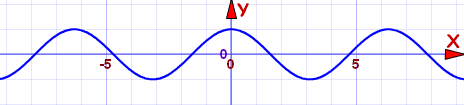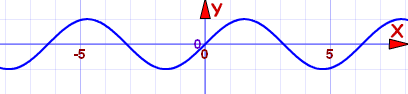# 偶函数与奇函数

## 偶函数

f(x) = f(−x) ，x 为任何值，−f(x) = f(−x)，x 为任何值，## 非奇也非偶## 偶还是奇？

### 例子：f(x) = x/(x2−1) 是偶函数、奇函数、或两个都不是？

−x 代入看看：

 代入 "−x"： f(−x) = (−x)/((−x)2−1) 简化： = −x/(x2−1) = −f(x)

f(−x) = −f(x)，所以它是奇函数

## 特别特性

• 两个偶函数的和是偶函数。
• 两个奇函数的和是奇函数。
• 偶函数和奇函数的和不是偶函数，也不是奇函数（除非其中一个函数是零）。

• 两个偶函数的积是偶函数。
• 两个奇函数的积是偶函数。
• 偶函数和奇函数的积是奇函数。